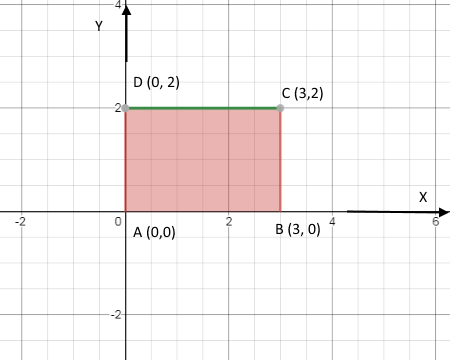# Find the absolute maximum and minimum values of f on the set D. f(x,y) = x^{4} + y^{4} - 4xy + 6,...

## Question:

Find the absolute maximum and minimum values of {eq}f {/eq} on the set {eq}D {/eq}.

{eq}f(x,y) = x^{4} + y^{4} - 4xy + 6, \quad\quad D = \{(x,y) \left| \right. 0 \leq x \leq 3,\;\; 0 \leq y \leq 2\} {/eq}

## Comparison of function using Calculus:

If we want to compare {eq}f(x) \ \ \text{and} \ \ g(x) {/eq} consider a function {eq}\phi(x) = f(x) - g(x) {/eq} or {eq}\phi(x) = g(x) - f(x) {/eq} and check whether {eq}\phi(x) {/eq} is increasing or decreasing in given domain of {eq}f(x) \ \ \text{and} \ \ g(x) . {/eq}

{eq}\hspace{30mm} \displaystyle{ f(x,y) = x^4 + y^4 - 4xy + 6 \\ f_{x} = 4x^3 - 4y \\ f_{y} = 4y^3 - 4x } {/eq}

Now to find the critical points solve the system:

{eq}\hspace{30mm} \displaystyle{ 4x^3 - 4y = 0 \\ 4y^3 - 4x = 0 } {/eq}

The real solution of this system is:

{eq}\hspace{30mm} \displaystyle{ (0,0) , \ \ (1,1) \ \ \text{and} \ \ (-1,-1) } {/eq}

Hence the critical points are: {eq}(0,0) , \ \ (1,1) \ \ \text{and} \ \ (-1,-1). {/eq}

To find the absolute maxima we also need to check the function at the boundary points of the given domain. Therefore the region of the given domain {eq}D {/eq} is given below in figure:Therefore the boundary points are {eq}A \ (0,0) , \ \ B \ (3,0) , \ \ C \ (3,2) \ \ \text{and} \ \ D \ (0,2) {/eq}

Therefore the value of function at these critical and boundary points is given below:

{eq}\text{At} \ \ (0,0) \ \ f(x,y) = 6 \\ \text{At} \ \ (1,1) \ \ f(x,y) = 4 \\ \text{At} \ \ (-1,-1) \ \ f(x,y) = 4 \\ \text{At} \ \ (3,0) \ \ f(x,y) = 87 \\ \text{At} \ \ (3,2) \ \ f(x,y) = 79 \\ \text{At} \ \ (0,2) \ \ f(x,y) = 22 \\ {/eq}

Therefore the given function has absolute maxima at {eq}(3,0) {/eq} in the given region. And has absolute minima at {eq}(1,1) \ \ \text{and} \ \ (-1,-1). {/eq}